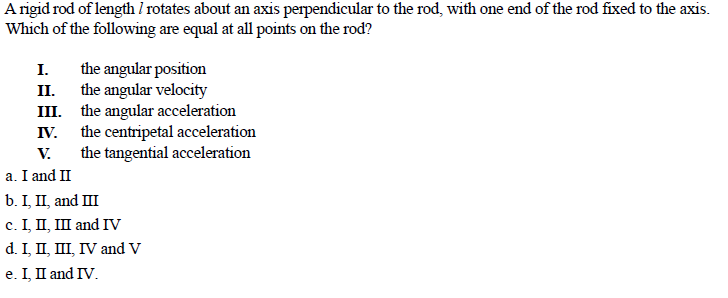# A rigid rod of length / rotates about an axis perpendicular to the rod, with one end of the rod fixed to the axis. Which of the following are equal at all points on the rod? I. the angular position II. the angular velocity III. the angular acceleration IV. the centripetal acceleration V. the tangential acceleration a. I and II c. 1. П. III and IV d.1, Π. Ш, IV and V

Questionhelp_outlineImage TranscriptioncloseA rigid rod of length / rotates about an axis perpendicular to the rod, with one end of the rod fixed to the axis. Which of the following are equal at all points on the rod? I. the angular position II. the angular velocity III. the angular acceleration IV. the centripetal acceleration V. the tangential acceleration a. I and II c. 1. П. III and IV d.1, Π. Ш, IV and V fullscreen